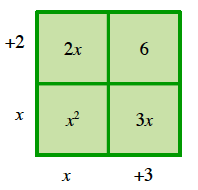Home > CCG > Chapter 2 > Lesson 2.2.4 > Problem2-95

2-95.

Multiply the expressions below. Then simplify the result, if possible. Homework Help ✎

Look at the Math Notes box in Lesson 2.2.2.

1. $3x(5x+7)$

2. $(x+2)(x+3)$3. $(3x+5)(x−2)$

4. $(2x+1)(5x−4)$

$10x^2−3x−4$